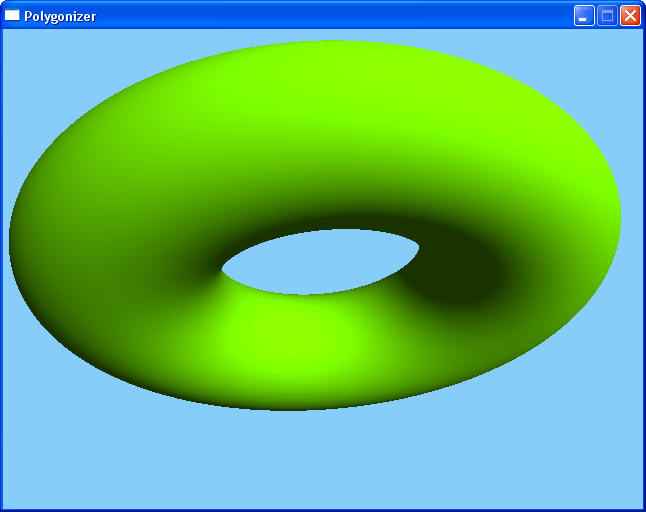# Polygonizer

Jules Bloomenthal wrote an article about an implicit surface polygonizer. I've ported his C program to Lisp. If you want to execute the polygonizer, you'll need lispbuilder-sdl, cl-opengl and optionally the Infix package for easier entering mathematical formulas.

## How it works

The polygonizer calculates the surface of a function. A point is on the surface of a function if the function value for this point is 0. A simple example is a torus:

```(defun torus (x y z)
"a torus"
(let* ((major 2.5)
(minor 1.2)
(x2 (* x x))
(y2 (* y y))
(z2 (* z z))
(a (+ x2 y2 z2 (* major major) (- (* minor minor)))))
(- (* a a) (* 4.0 (* major major) (+ y2 z2)))))
```

Then we define a callback function, which is called by the polygonizer for every triangle it creates:

```(defun triangle (p1 p2 p3 n1 n2 n3)
(incf *triangle-count*)
(gl:begin :triangles)
(gl:normal (point-x n1) (point-y n1) (point-z n1))
(gl:vertex (point-x p1) (point-y p1) (point-z p1))
(gl:normal (point-x n2) (point-y n2) (point-z n2))
(gl:vertex (point-x p2) (point-y p2) (point-z p2))
(gl:normal (point-x n3) (point-y n3) (point-z n3))
(gl:vertex (point-x p3) (point-y p3) (point-z p3))
(gl:end))
```

After initializing OpenGL, we call the polygonizer:

```(polygonize #'torus 0.10 100 0.0 0.0 0.0 #'triangle TET)
```

The output:For more complex functions, like this one from the paper:it is easier to use the Infix package, if you are not a hardcore Lisp programmer, to write it like this:

```(defun jack (x y z)
#I"(1/(x^^2/9+4*y^^2+4*z^^2)^^4
+1/(y^^2/9+4*x^^2+4*z^^2)^^4
+1/(z^^2/9+4*y^^2+4*x^^2)^^4
+1/((4*x/3-4)^^2+16*y^^2/9+16*z^^2/9)^^4
+1/((4*x/3+4)^^2+16*y^^2/9+16*z^^2/9)^^4
+1/((4*y/3-4)^^2+16*x^^2/9+16*z^^2/9)^^4
+1/((4*y/3+4)^^2+16*x^^2/9+16*z^^2/9)^^4)^^(-1/4)-1")
```

This produces the following surface:17. April 2006, Frank Buß